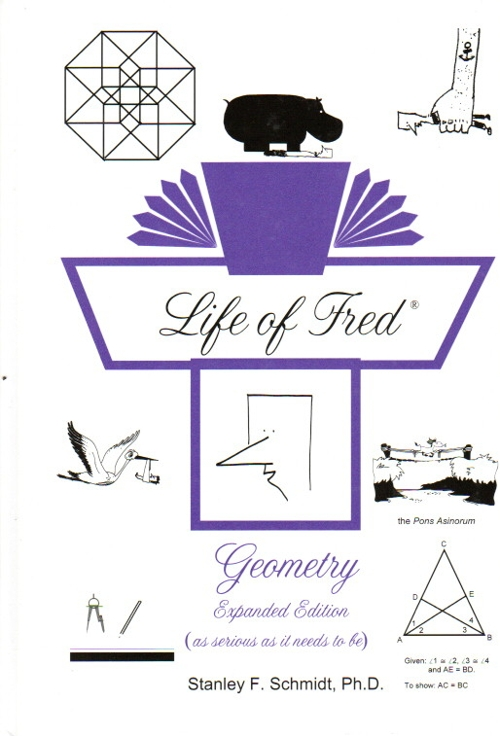Life of Fred Geometry \$49 : Thirteen regular chapters and six bonus honors chapters. Points and lines, Definition of when one point is between two other points, Definition of a triangle, Midpoint, Proof of a theorem in paragraph form, Tangent and secant lines, Area and volume formulas, Undefined terms, Hypothesis and conclusion, Contrapositives, Postulates, Euclid’s The Elements, Rays, Acute, obtuse, and right angles, Congruent angles, Linear pairs, Vertical angles, Supplementary angles, Perpendicular lines, SSS, SAS, ASA, Isosceles triangle theorem, Pons Asinorum, Parallel lines, Skew lines, Indirect proofs, Attempts to prove the parallel postulate, Exterior angles, Two proofs of the exterior angle theorem, Hypotenuse-leg theorem, Medians, Quadrilaterals: parallelogram, rhombus, trapezoid, kite, rectangle, square, Honor’s Problem of the century: If two angle bisectors are congruent when drawn to the opposite sides, then the triangle is isosceles, Definition of a polygon, Proofs of all the area formulas given only the area of a square (This is hard. Most books start with the area of a triangle as given.) Proofs of the Pythagorean theorem, Geometries with only three points, Similar triangles, Five manipulations of proportions, Angle bisector theorem, Symbolic logic, Truth tables, Circles, Inscribed angle theorem, Definition of a limit of a function, Inductive and deductive reasoning, Golden rectangles, 46 Ruler-and-compass constructions, Non-euclidean geometries, Solid geometry, Euler’s theorem, Geometry in high dimensions, Stereochemistry and homochirality, Complete chart up to the 14th dimension, Proofs using analytic geometry, Proof that a right angle is congruent to an obtuse angle using euclidean geometry, Flawless (modern) geoemtry which was invented about a hundred years ago. ISBN: 978-1-937032-21-0, hardback, 560 pages. \$49